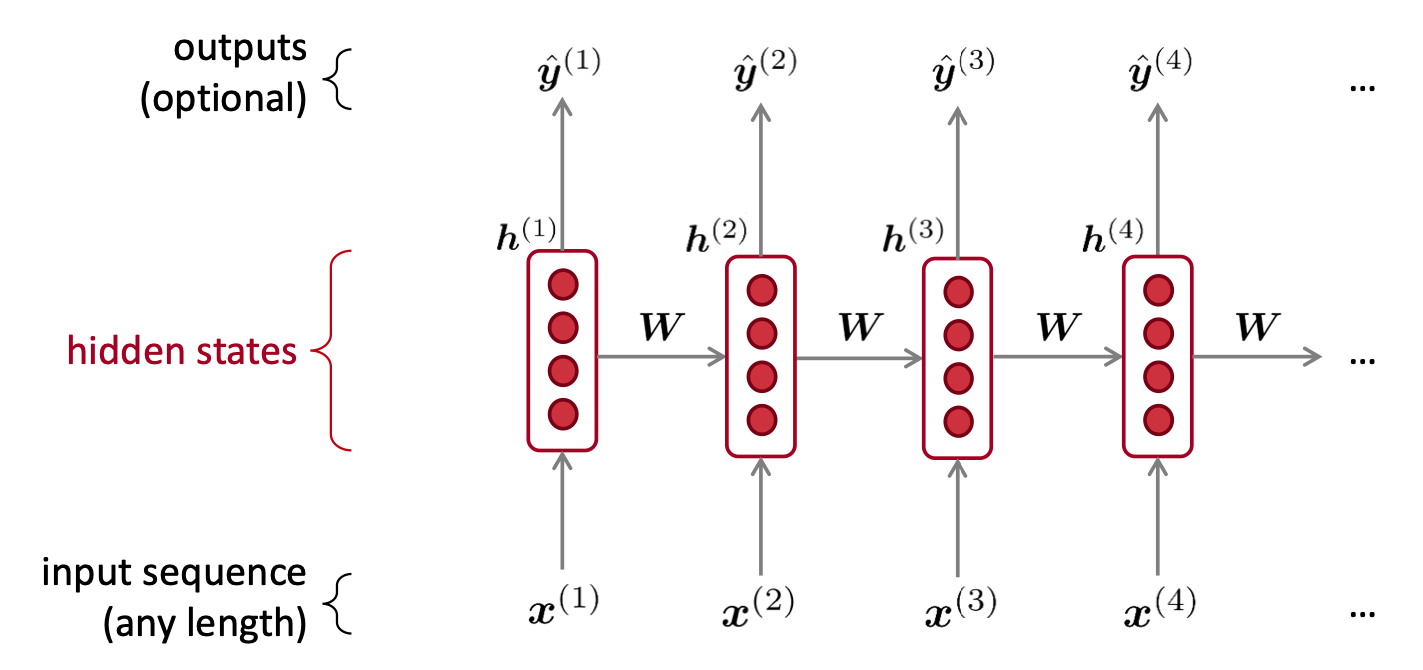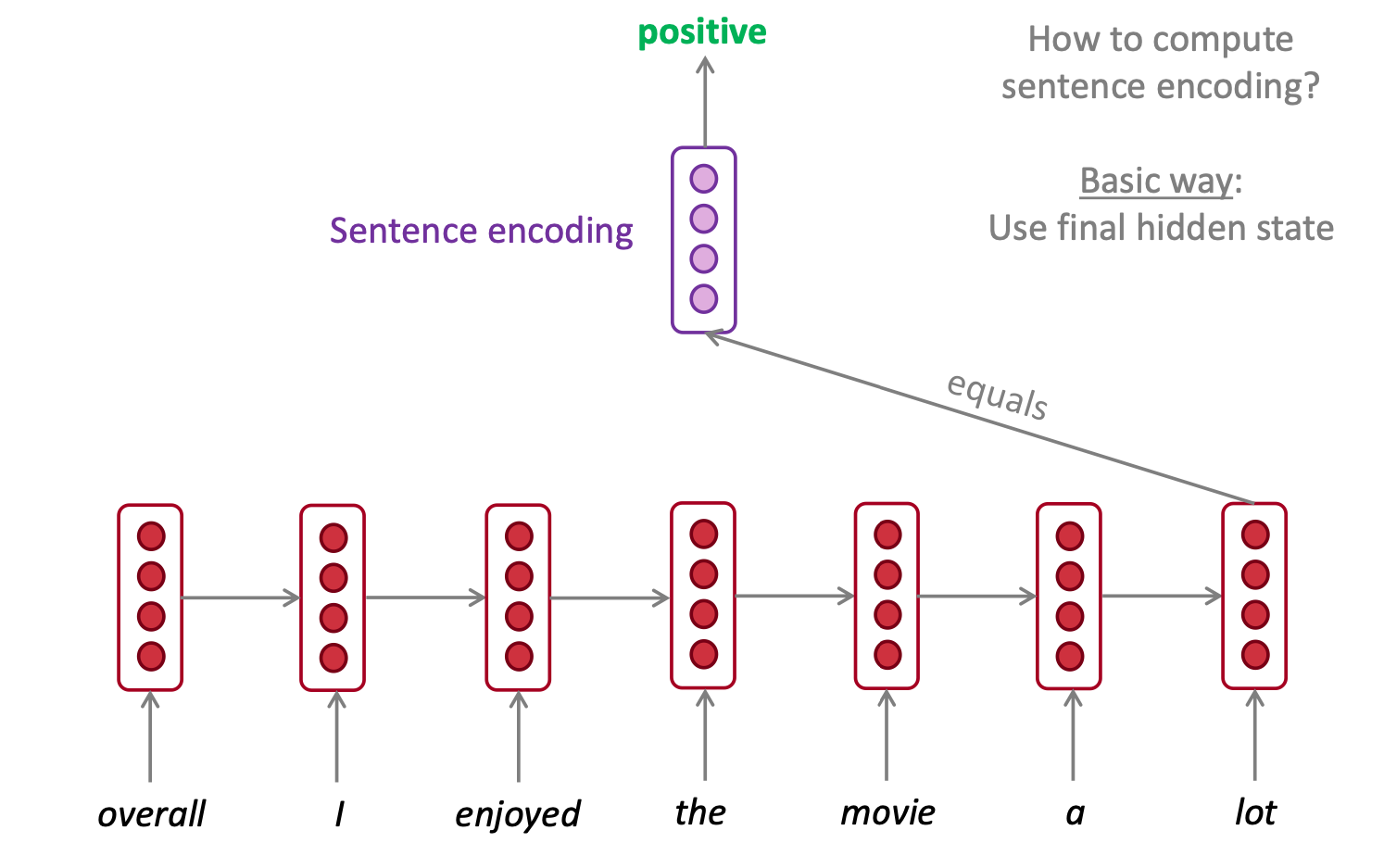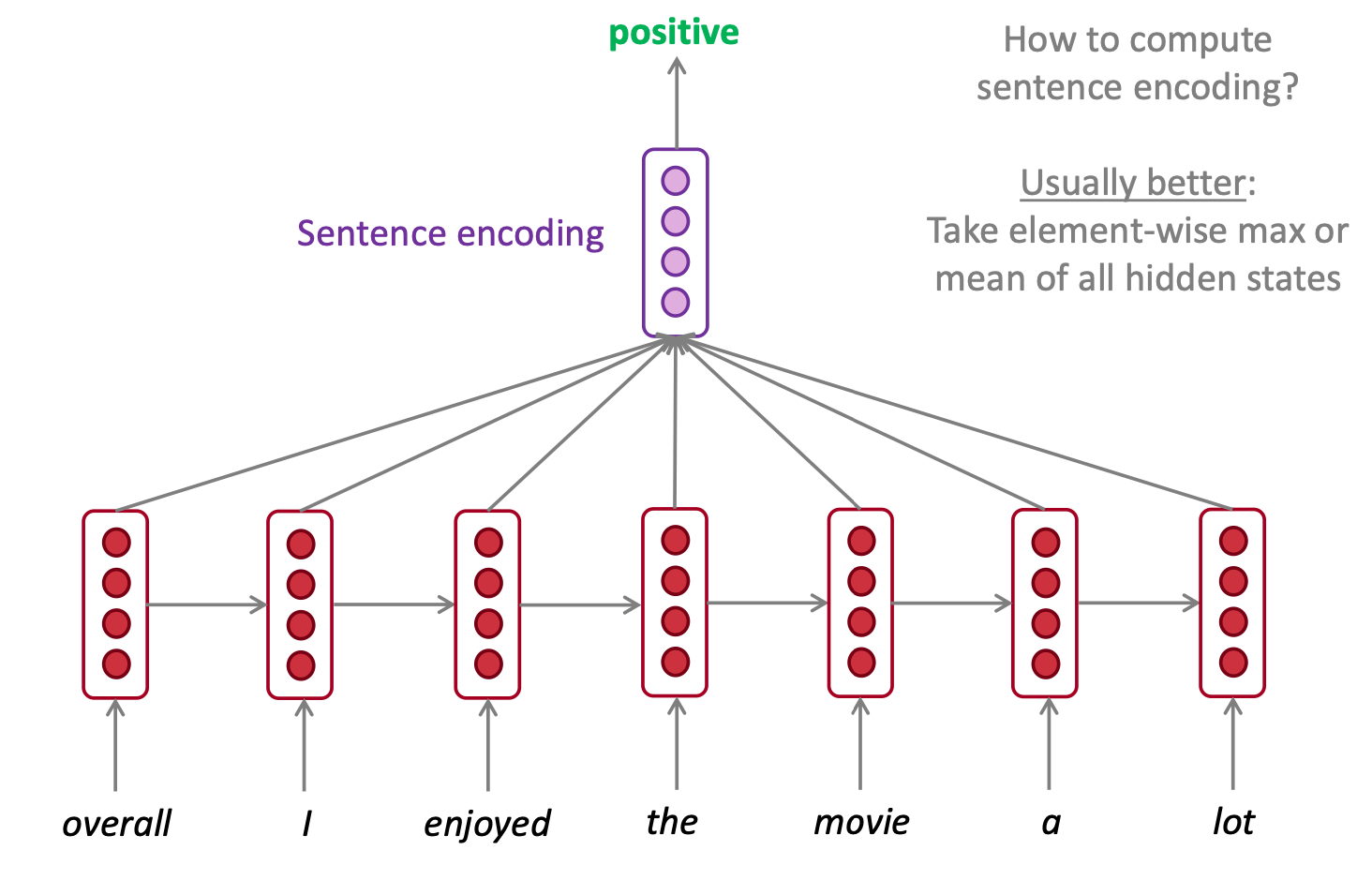# Introduction

In this NLP getting started challenge on kaggle, we are given tweets which are classified as 1 if they are about real disasters and 0 if not. The goal is to predict given the text of the tweets and some other metadata about the tweet, if its about a real disaster or not.
In this part 7 for building RNNs, I will use the data module generated in Part 5 to create a RNN models to predict if the tweet is about a real disaster. Following up from the previous Part 6 about multi-layer perceptron model, I will generate the prediction output of this model on the validation set and compare results.

# Classifier

I will be using the pytorch-lightning framework to build a classifier class.
Let’s follow the same overall flow of modelling that we had in Part 6. The training pipeline will have the following components -

• Network architecture
• Loss function
• Optimizer
• Forward Pass
• Training Step / Val Step / Test Step

Note that the Loss Function, the Optimizer and the training/val/test steps remains the same as our Multilayer Perceptron from Part 6, so I will only discuss the remaining 2 components in this part.

## Network Architecture

The essential idea of a Recurrent Neural Network is to process the words (tokens) in an input sentence sequentially, by applying the same weights W repeatedly to the output of previous word. The output at each processed word is called the hidden state. It looks like the following pictorial representation.Recurrent Neural Network Pictorial representation from https://web.stanford.edu/class/archive/cs/cs224n/cs224n.1194/slides/cs224n-2019-lecture06-rnnlm.pdf

### Vanilla RNNs for Sentence Classification

In our usecase, we will use the above vanilla RNN to create a “Sentence Encoding” i.e. a vector of values that represent the given sentence and then pass that vector through another linear layer to make our final binary prediction of whether the given tweets is about a real disaster or not.

The network architecture looks as follows.

``````import pytorch_lightning as pl

class DisasterTweetsClassifierRNN(pl.LightningModule):

def __init__(self, rnn_hidden_size, num_classes,
dropout_p, pretrained_embeddings, learning_rate,
num_layers, bidirectional, aggregate_hiddens, aggregation_func='max'):
super().__init__()

self.save_hyperparameters('num_classes', 'dropout_p', 'learning_rate',
'rnn_hidden_size', 'num_layers', 'bidirectional',
'aggregate_hiddens', 'aggregation_func'
)

embedding_dim = pretrained_embeddings.size(1)
num_embeddings = pretrained_embeddings.size(0)

self.emb = torch.nn.Embedding(embedding_dim=embedding_dim,
num_embeddings=num_embeddings,
_weight=pretrained_embeddings)

self.rnn = torch.nn.RNN(embedding_dim, rnn_hidden_size, num_layers=num_layers, bidirectional=bidirectional)
rnn_output_size = rnn_hidden_size
if bidirectional:
rnn_output_size = rnn_hidden_size * 2
self._dropout_p = dropout_p
self.fc1 = torch.nn.Linear(rnn_output_size, rnn_hidden_size)
self.fc2 = torch.nn.Linear(rnn_hidden_size, num_classes)

self.loss = torch.nn.CrossEntropyLoss(reduction='none')``````
• We are logging the hyperparameters of our network and initializing our embedding layer with the pretrained embeddings.
• We then create our vanilla RNN object with the required parameters.
• One parameter that gets passed to the RNN object is the number of layers. In order to generate more predictive power we can stack multiple RNNs on top of each other, this parameter configures the number of those stacked RNN layers.
• We also pass the `bidirectional` flag to the RNN object. Like we discussed earlier, RNN processes input sentence sequentially, we can process the sentence from beginning to end or end to beginning. Usually, processing from both the directions and using the concatenated vector of both directions leads to higher predictive power.
• Finally we have 2 linear layers which transform the RNN output into our 2 layer output which can be used to calculate cross entropy loss.

### Gated Recurrent Units (GRU) for Sentence Classification

Vanilla RNNs suffer from the problem of Vanishing Gradients. In simple words, the basic RNNs discussed above are more impacted by near word effects instead of words which are far away. So, for sentences with longer length, at each RNN step, the model is only able to carry information from the previous few words and not from the words which are farther away but may have more relevance to the word in current step.
The issue mainly arises because we completely modify hidden state and weights at each step based on the currently incoming word and the hidden state vector becomes an information bottleneck. GRUs try to fix this problem by controlling how much of the previous hidden vector is forgotten and how much of it is kept/updated. Another popular variant to fix this problem is the LSTM (Long Short Term Memory) network which tries to keep a separate vector (cell state) as “memory” to hold information over long sequences. However, I am using GRU in my work in favour of limited compute power.

This lecture by Abigail See at Stanford is the best resource IMO to understand the issues with Vanilla RNNs and how GRUs and LSTMs work to try and resolve them.

For our purposes, we create the GRU network as follows -

``````import pytorch_lightning as pl

class DisasterTweetsClassifierGRU(DisasterTweetsClassifierRNN):
def __init__(self, rnn_hidden_size, num_classes,
dropout_p, pretrained_embeddings, learning_rate,
num_layers, bidirectional, aggregate_hiddens, aggregation_func='max'):
super().__init__(rnn_hidden_size, num_classes,
dropout_p, pretrained_embeddings, learning_rate,
num_layers, bidirectional, aggregate_hiddens, aggregation_func=aggregation_func)

embedding_dim = pretrained_embeddings.size(1)
self.rnn = torch.nn.GRU(embedding_dim, rnn_hidden_size, num_layers=num_layers, bidirectional=bidirectional)``````
• Note that this class has the vanilla RNN class as a parent so it borrows almost all the implementation from there.
• The only difference in this GRU network is the usage of `torch.nn.GRU` instead of `torch.nn.RNN` in order to process the input sentences.

## Forward Method

The simplest and most basic way to create a sentence encoding using the above RNN is by just using the final hidden state of the sentence as the Sentence Encoding. Pictorially it looks like this.Recurrent Neural Network used for Sentence Classification Basic - Pictorial representation from https://web.stanford.edu/class/archive/cs/cs224n/cs224n.1194/slides/cs224n-2019-lecture06-rnnlm.pdf

A usually better way is to do an element wise aggregation (max or mean) of all hidden states as theoretically it will contain information from all parts of the sentence while contributing to sentence encoding.Recurrent Neural Network used for Sentence Classification Aggregation - Pictorial representation from https://web.stanford.edu/class/archive/cs/cs224n/cs224n.1194/slides/cs224n-2019-lecture06-rnnlm.pdf

The following forward method handles both of these usecases based on the given hyperparameters to the network.

``````class DisasterTweetsClassifierRNN(pl.LightningModule):
def forward(self, batch, batch_lengths):
x_embedded = self.emb(batch)
batch_size, seq_size, feat_size = x_embedded.size()
x_embedded = x_embedded.permute(1, 0, 2)

initial_hidden = self._initialize_hidden(batch_size)

hidden_all, _ = self.rnn(x_embedded, initial_hidden)

hidden_all = hidden_all.permute(1, 0, 2)
if self.hparams.aggregate_hiddens:
features = self.element_wise_aggregate(hidden_all, batch_lengths, self.hparams.aggregation_func)
else:
features = self.column_gather(hidden_all, batch_lengths)

int1 = torch.nn.functional.relu(torch.nn.functional.dropout(self.fc1(features),
p=self._dropout_p))
output = self.fc2(torch.nn.functional.dropout(int1, p=self._dropout_p))
return output``````

In the forward pass through the network, we first generate the embeddings for our batch and initialize a default hidden layer of all zeroes. The embeddings and initial hidden layer are passed to the RNN to get all the hidden layers. Next, based on our `aggregate_hiddens` boolean hyperparameter, we either perform an element wise aggregation on all hidden layers if the hyperparameter is true or just gather the final hidden layer for each tweet in the batch, if the hyperparameter is false.
This final vector then gets passed through our `fc` fully connected layers and generates the output which gets returned.

# The Training Routine

The training routine for these networks remains almost exactly the same as the one in Part 6. Instead of instantiating an object of class `DisasterTweetsClassifierMLP`, we create objects of class `DisasterTweetsClassifierRNN` or `DisasterTweetsClassifierGRU`.

# Results

Model Bidirectional Aggregate Hidden Layers Aggregation Function Accuracy F-1 Score
Vanilla RNN No No NA 0.7747589833479404 0.7233584499461788
GRU No No NA 0.761612620508326 0.7124735729386892
Vanilla RNN Yes No NA 0.7502191060473269 0.6958377801494131
GRU Yes No NA 0.7712532865907099 0.7096774193548387
Vanilla RNN Yes Yes Max 0.7817703768624014 0.7254685777287762
GRU Yes Yes Max 0.782646801051709 0.7268722466960353
Vanilla RNN Yes Yes Mean 0.7703768624014022 0.7127192982456141
GRU Yes Yes Mean 0.7922874671340929 0.7392739273927393

The above table shows the results for the model’s performance on our validation set when trained with different combinations of hyperparameters (and a modest number of epochs, given the compute power on my mac). Also I have stack 3 layers of RNNs in all above experiments (i.e. `num_layers=3`) A few things to note -

• GRUs in general perform better than Vanilla RNNs for our usecase.
• Bidirectionality performs poorer for our given problem.
• Aggregating hidden layers is better than just using the last hidden layer as the sentence encoding.
• Bidirectional GRU with Mean aggregation of hidden layers gives the best result of `0.7392739273927393` F1 Score. This F1 score is lower in comparison to the multilayer perceptron that we built in Part 6 and also much lower than the XGBoost tree we built in Part 4.

# Summary

In this part of the series, we built a few Recurrent Neural Networks to predict if a tweet is about a real disaster using the PyTorch Lightning framework. We built this on top of the Lightning Data Module that we discussed in the previous post of this series. We can see that we get a good performance from this network and can hopefully with further hyperparameter tuning improve it. The full code for this post can be viewed on my github here. In the next part, I will use the Transformer Architecture based pre-trained models like BERT to generate predictions for our usecase.